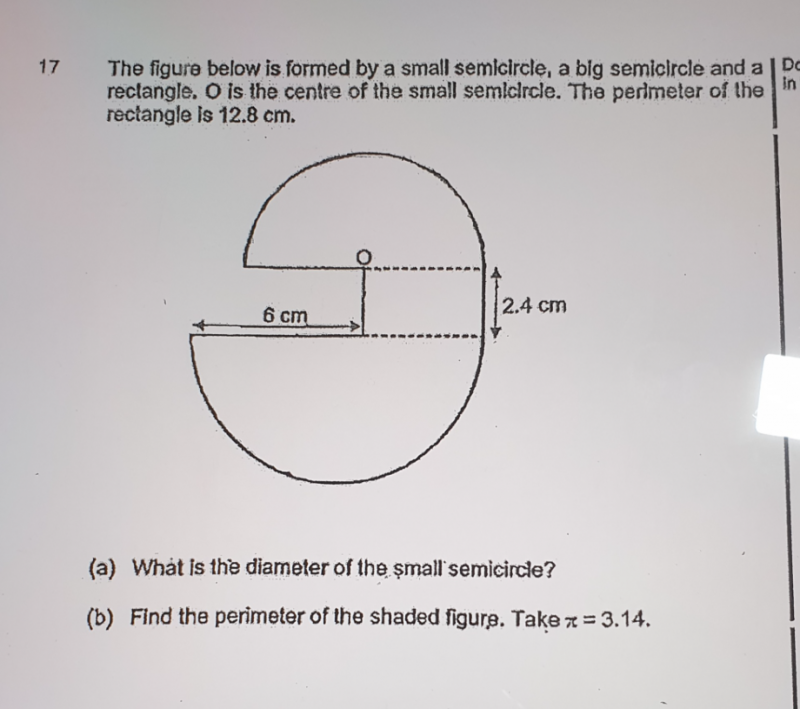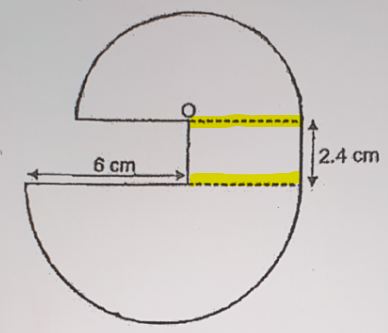# QuestionHi, Need assistance to solve this question.

Thank You.

(a) Perimeter of rectangle = 12.8

Diameter of small circle =  12.8 – 2(2.4) = 8cm

(b) Radius of small circle, r = 4

Radius of big circle, R = (6+4)/2 = 5

2 vertical lines = 2(2.4) = 4.8   and 2 horizontal lines = 6 + 4 = 10

Perimeter of the figure = πr + πR + 4.8 + 10    (semi circle circumference = 2πr / 2 = πr)

= (3.14)(4) + (3.14)(5) + 14.8  = 43.06cm

0 Replies 1 Like

a. The perimeter of the rectangle is 12.8cm, so the length of the rectangle = 12.8/2 – 2.4 = 4cm. That is also the radius of the small circle (as shown by yellow bar in diagram below). So diameter of small semicircle is 2 x 4 = 8cm.

b. I don’t see any shaded figure. I presume you meant the perimeter of the whole figure. First, we know the radius of big semicircle is (6 + 4)/2 = 5cm.

So the perimeter  of the whole figure

= pi x radius_small + pi x radius_big + 4 + 6 + 2 x 2.4

= 3.14 x (4 + 5) + 14.8 = 43.06cm.0 Replies 1 Like